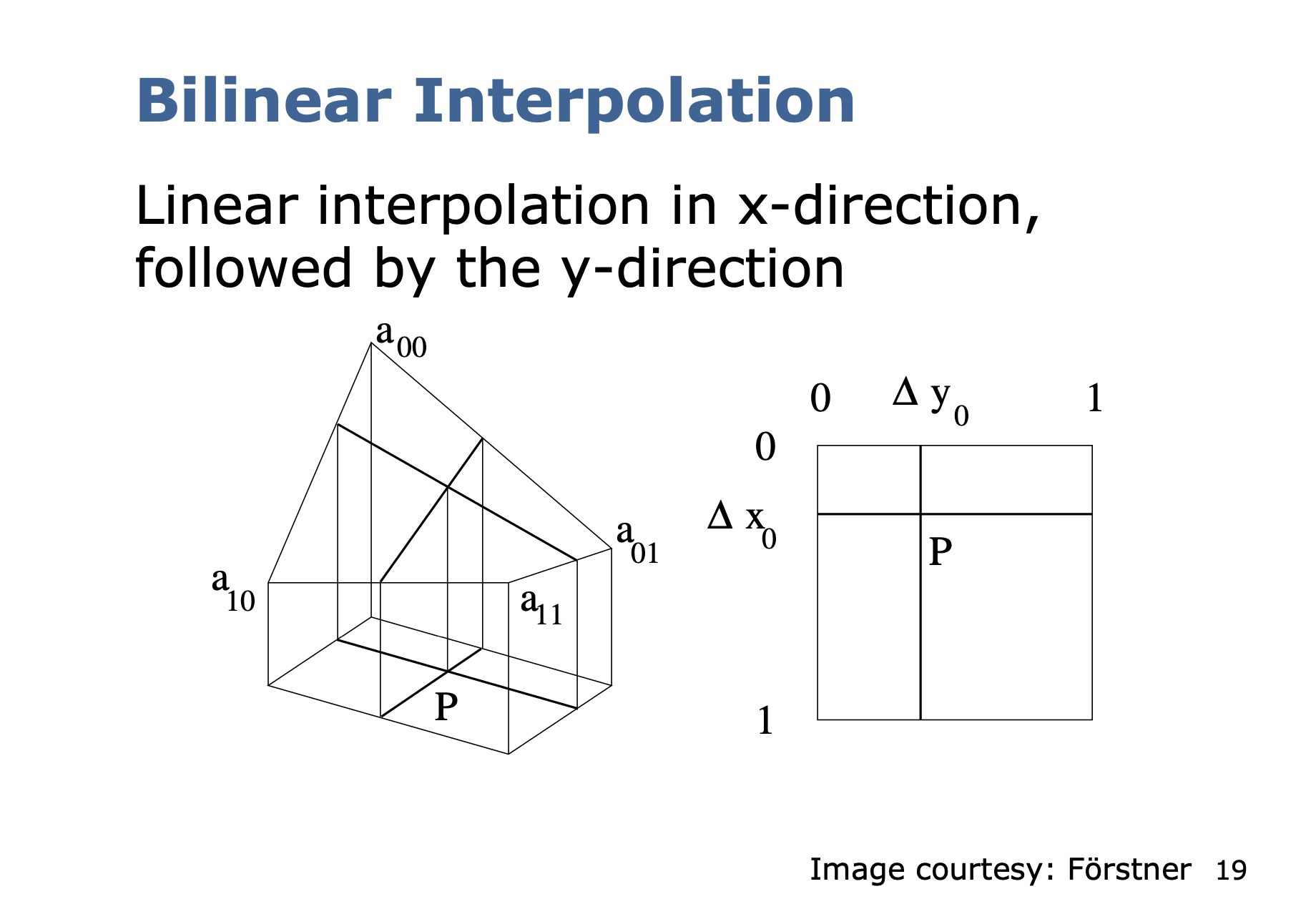Image Interpolation

# Bilinear Interpolation §

Heard Jack Zhang from NVIDIA say this term while he was working on ESS.

Resources

notes from Cyrill Stachniss.

##### Bilinear Interpolation §

This is intuitive. To calculate the new intensity value, compute a weighted average of the 4 neighbouring pixel intensity values.\begin{align} b(x,y) = &a_{00} (1- \Delta x)(1- \Delta y) + a_{01}(1-\Delta x)\Delta y \\ &+ a_{10} \Delta x (1 - \Delta y) + a_{11} \Delta x \Delta y \end{align}

• Where are the 4 neighbouring pixel intensity values

Normalized

Notice that things seem to be normalized between 0 and 1 here. I don’t know how I am supposed to do this in practice.

Cyrill Stachniss likes to write this more compact form

However, be careful since doesn’t correspond to .

How am I supposed to determine ?

You need to compare the original equation term by term.

c_{01} &= a_{01} - a_{00} \\ c_{10} &= a_{10} - a_{00} \\ c_{11} &= a_{11} - a_{10} - a_{01} + a_{00} \\ \end{align} ![[attachments/Screenshot 2023-10-08 at 1.38.46 PM.png]]